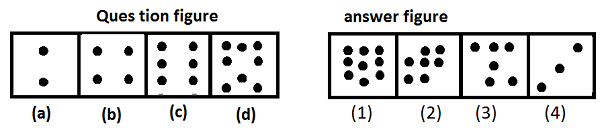# Figure Series Online Quiz

Following quiz provides Multiple Choice Questions (MCQs) related to Figure Series. You will have to read all the given answers and click over the correct answer. If you are not sure about the answer then you can check the answer using Show Answer button. You can use Next Quiz button to check new set of questions in the quiz.Q 1 - Select a figure from answer set which must continue the series of question figure.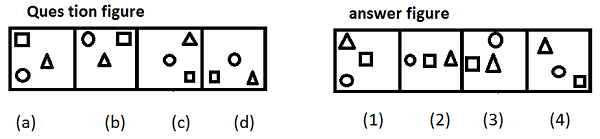### Explanation

Each figure remains at centre for two step and followed by other in next step, other figure are arranging themselves in clock rotation.

Q 2 - Select a figure from answer set which must continue the series of question figure.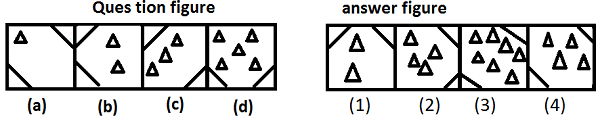### Explanation

In each step one line from corner moves to other corner anti-clockwise and number of triangles are increasing as per consecutive prime number.

Q 3 - Which figure must be placed in place of question mark?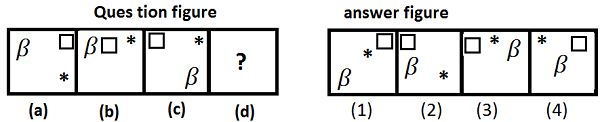### Explanation

The symbols are changing their position alternately as per following figure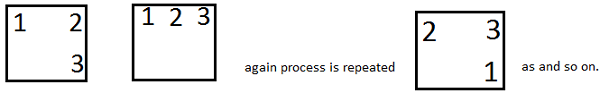Q 4 - Which figure is not following the sequence?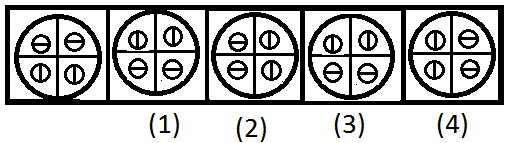### Explanation

The small circles are spinning about their axis in one step and changing position in clockwise direction in another step hence it is clear that figure 3 and 4 must interchange their position hence figure 3 is the answer.

Q 5 - Find the answer figure from alternatives which are given numbered from 1 to 4 by using the sequence of set of figures given in question figure.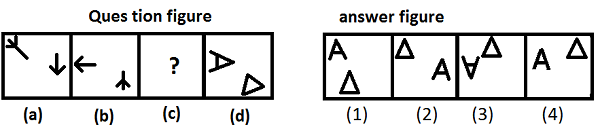### Explanation

The figure at top left corner moves to bottom right corner by turning through 135° clock wise direction while other figure is turning through 90° in clock wise direction and moves to left side.

Q 6 - Find the answer figure from alternatives which are numbered from 1 to 4 by using the sequence of set of figures given in question figure.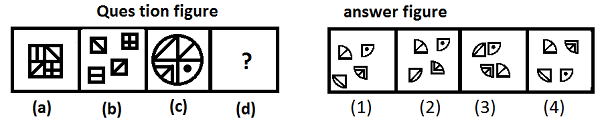### Explanation

The whole figure is divided into quarters and arranged as per the following figure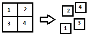. The first quarter is rotating through an angle of 90° anti-clockwise.

Q 7 - Which figure in the answer figure will replace the question mark?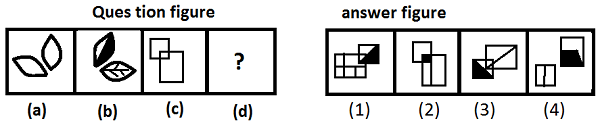### Explanation

Each figure contains two parts. In next step one gets modified and other is shaded half, also followed by rotation of figure through an angle of 90° clockwise.

Q 8 - Which figure is different from others?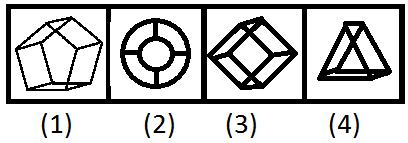### Explanation

Except figure (2), other figures are 3D view of various geometric figures.

Q 9 - Which figure is different from others?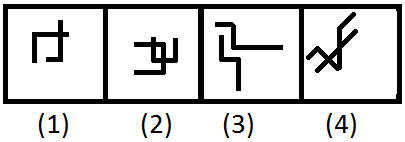### Explanation

In other three figures, lines are intersecting each other.

Q 10 - Which figure is similar to question figure?